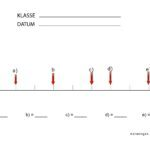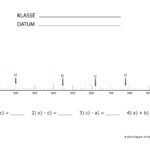# Number line - exercises

On this page you will find some exercise sheets for calculating on a number line together with clear instructions on how to calculate the distances on a number line. Calculating on the number bar illustrates the distances and relationships between numbers in the respective number range.

## Calculate with the number line

Important rules: the distances between the numbers are always the same and the further you go to the right on the number line, the larger the number becomes. Since processes are shown in a similar way on a timeline, one should not deviate from this left-to-right system.

You can find short instructions for calculating on the number line at the end of this page. A click on a drawing opens the page with the selected number line:Number line to 100Number line to 100Number line to 1000Number line to 1000Number line up to 1000 less scalingNumber line up to 1000 less scalingNumber line up to 2000 without scalingNumber line up to 2000 without scalingCalculate scalingCalculate scalingCalculate on the number line up to 100Calculating on the number line up to 1000

### Calculate distances on the number line

If you don't want to find out the distances between the marks by trying, you can find out the distances by the following calculations:

Example 1 - when the number line starts at zero1. Find the total length of the number line. This corresponds to the last number (500)
2. Count the number of sections of the same size (higher bars) (5)
3. Divide the length of the number line by the number of sections (500: 5 = 100)
4. So the higher distances are 100, 200, 300 and 400
5. We calculate the smaller distances in the same way: total length of the partial area (100) divided by the number of sections (10) = 10
6. The small distances are als0 10, 20, ..., 90)

Example 1 - when the number line does not start at zero1. Find the total length of the number line. This corresponds to the subtraction from the last minus the first number
(700 -300 = 400)
2. Count the number of sections of the same size (higher bars) (4)
3. Divide the length of the number line by the number of sections (400: 4 = 100)
4. The higher distances are 100, 200, 300 and 400. Because the number line does not start with zero, you have to add 100 to the first number. The other distances in our example are therefore 300 + 100 = 400, 500 and 600)
5. We calculate the smaller distances again in the same way: total length of the sub-area (100) divided by the number of sections (10) = 10
6. The small distances are als0 10, 20, ..., 90)

Additional info: even if the terms number line and number line are used arbitrarily in school lessons, a number line actually only includes positive numbers (and therefore always has zero as the smallest possible number), while a number line can also show negative numbers. But, as I said, it is very often mixed up.

Do you have any questions, suggestions or criticism? Please do not hesitate to contact us. Are you looking for more templates for school lessons or to practice arithmetic on the number line with your children at home? Feel free to contact us for new ideas!